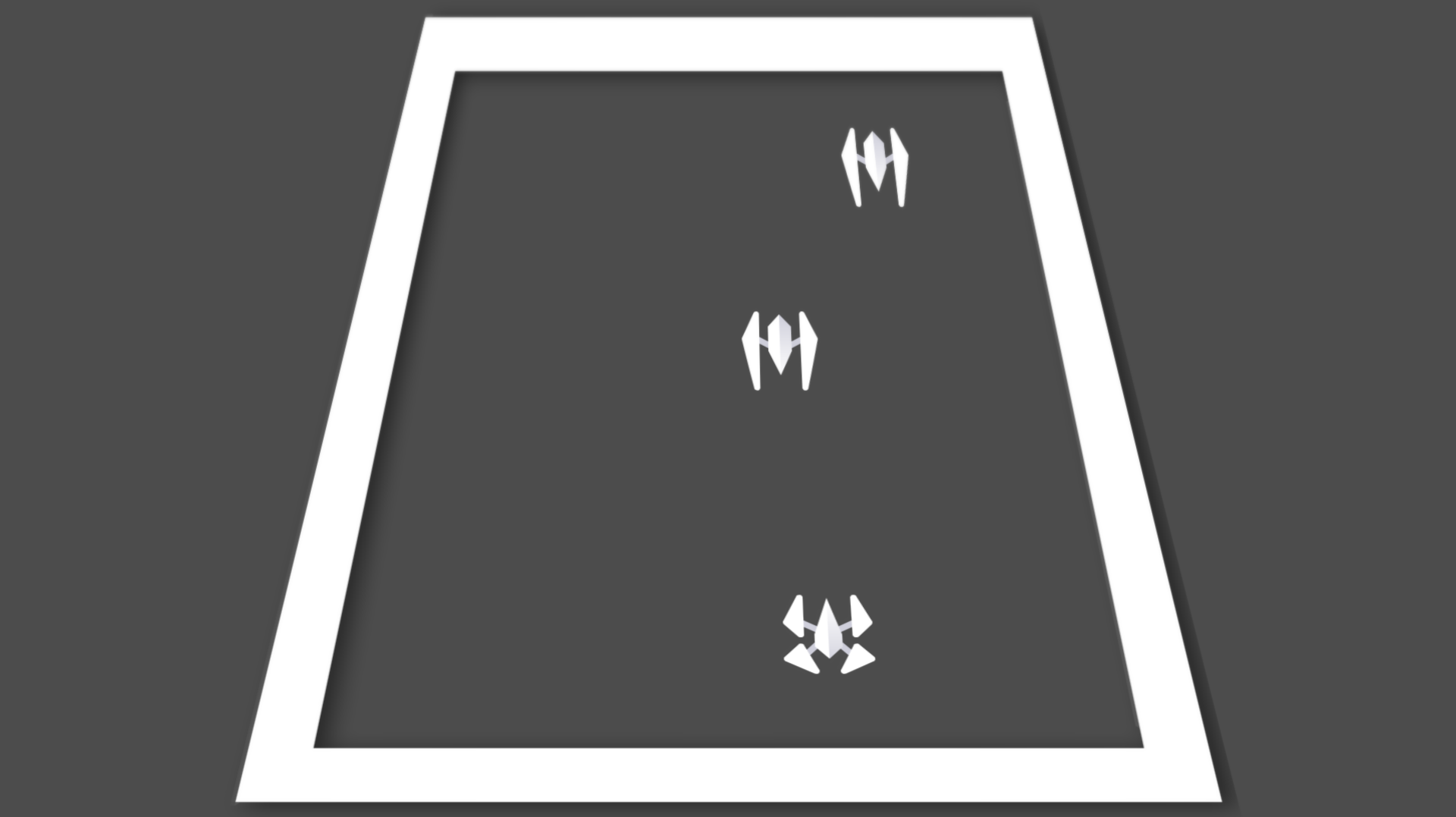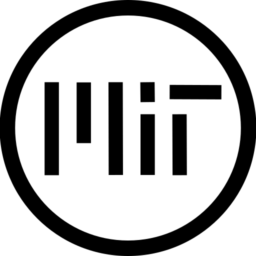Credit to this guy’s blog because the code is basically entirely his, I just converted it to Godot.

Just add the shader code to a `ColorRect` (or any control node with a texture) and set its anchor to full rect. Like this:

``````- CanvasLayer
``````

Then, in the shader parameters, change the `topright/topleft/bottomright/bottomleft` parameters to change the skew/perspective.

``````shader_type canvas_item;

uniform vec2 topleft = vec2(0.01, 0.0);
uniform vec2 topright;
uniform vec2 bottomleft;
uniform vec2 bottomright;

float _cross( in vec2 a, in vec2 b ) { return a.x*b.y - a.y*b.x; }

vec2 invBilinear( in vec2 p, in vec2 a, in vec2 b, in vec2 c, in vec2 d ) {
vec2 res = vec2(-1.0);

vec2 e = b-a;
vec2 f = d-a;
vec2 g = a-b+c-d;
vec2 h = p-a;

float k2 = _cross( g, f );
float k1 = _cross( e, f ) + _cross( h, g );
float k0 = _cross( h, e );

// if edges are parallel, use a linear equation.
if( abs(k2)<0.001 ) {
res = vec2( (h.x*k1+f.x*k0)/(e.x*k1-g.x*k0), -k0/k1 );
}
else {
float w = k1*k1 - 4.0*k0*k2;
if( w<0.0 ) return vec2(-1.0);
w = sqrt( w );

float ik2 = 0.5/k2;
float v = (-k1 - w)*ik2;
float u = (h.x - f.x*v)/(e.x + g.x*v);

if( u<0.0 || u>1.0 || v<0.0 || v>1.0 ) {
v = (-k1 + w)*ik2;
u = (h.x - f.x*v)/(e.x + g.x*v);
}
res = vec2( u, 1.0 - v );
}

return res;
}

void fragment(){
vec2 topleftUV = topleft / vec2(textureSize(TEXTURE,0));  // compensates for screensize ratio
vec2 toprightUV = vec2(1.0,0.0)+topright / vec2(textureSize(TEXTURE,0));
vec2 bottomrightUV = vec2(1.0,1.0)+bottomright / vec2(textureSize(TEXTURE,0));
vec2 bottomleftUV =vec2(0.0,1.0)+ bottomleft / vec2(textureSize(TEXTURE,0));

vec2 newUV = invBilinear(UV, topleftUV, toprightUV, bottomrightUV, bottomleftUV);

if (topleft.x == 0.0 || topright.x == 0.0) {
COLOR = texture(SCREEN_TEXTURE, UV);
}
else {
if (newUV == vec2(-1.0)){
COLOR = vec4(0.0);
}else {
COLOR = texture(SCREEN_TEXTURE, newUV);
}
}
}``````
###### Tags
perspective, screen, skew, warp

### Perspective Grid Remix March2021The shader code and all code snippets in this post are under MIT license and can be used freely. Images and videos, and assets depicted in those, do not fall under this license. For more info, see our License terms.Inline Feedbackssnesmocha
4 months ago

oh my god, thank you so muchsnesmocha
4 months ago

alright, after using the shader, the shader does not work and the shader itself isn’t being overlayed correctlysnesmocha
4 months ago

ok i figured out an easy fix to it, just change screen_space to texture 2dJUN
2 months ago